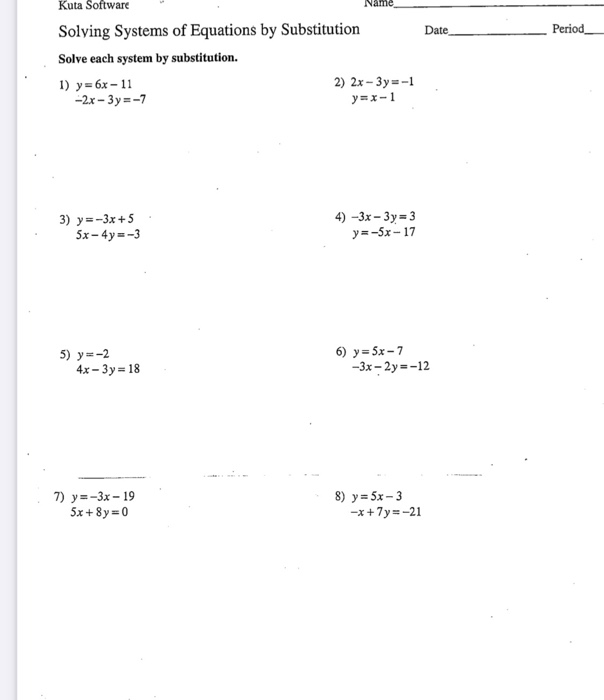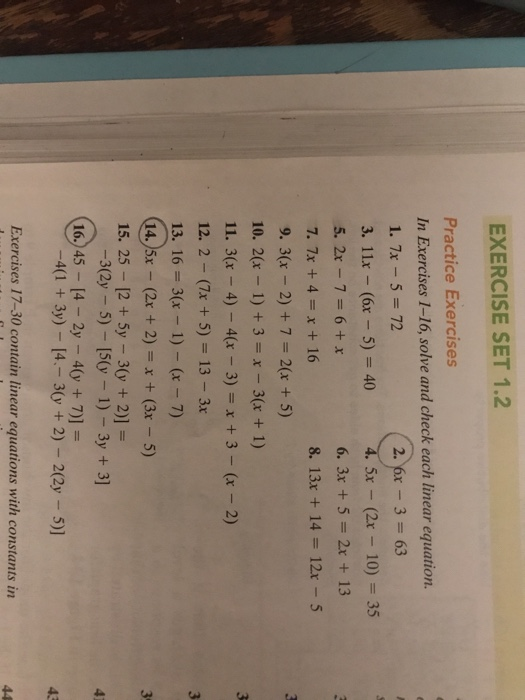# solve for x 1

solve for x

1. -5y+7=-18

2.-12=3a-5a

3. 2.5p=0.5p+14

4. 3x-7-+(-5x+8)

No what you should do is all adding/subtracting first then dividing/ multiplying.
2.5p=.5p+4

divide both sides by .5 p.

You will be left with 5p=4

Divide 4 by 5

Similar Homework Help Questions
• ### solve for X

solve for X ?1) 8-3x < 14-3x/-3 < 6/-3x=-22) 7x -3 > 4x +3 3x -3> 33x/3 >6/3 x>23) x-5<3 and 2x > -8 x<8 x>-44) x+ 7 <3 or 5x > 30 x<-4 x> 6are they correct..

• ### 2X^2+3X+1 d^2+8d+7 2a^2+5a+3 3x^2-x-4 2n^2+n-6 x^2+5x-14 5x^2-2x-7 7n^2+9n+2 3x^2+8x+4 7a^2-30a+8

2X^2+3X+1d^2+8d+72a^2+5a+33x^2-x-42n^2+n-6x^2+5x-145x^2-2x-77n^2+9n+23x^2+8x+47a^2-30a+8

• ### 2x-y=1 6x-3y=3 2

1. 2x-y=1 6x-3y=3 2. y=5x-1 y=5x+4 3. x+y=38 x=2y-25 4. -2x+5y=9 x+y=13 5. 2x+y=7 3x-y=3 6. y=3x+2 2x+y=-8 7.y=-x-3 y=-2x-8 8. yx+1 9. y=x y=2x-4 10. -2x+y=6 y=3x+9 11. y

• ### Name Date Period Kuta Software Solving Systems of Equations by Substitution Solve each system by substitution....Name Date Period Kuta Software Solving Systems of Equations by Substitution Solve each system by substitution. 1) y=6x-11 2) 2x - 3y = -1 -2x - 3y =-7 y=x-1 3) y=-3x + 5 5x - 4y=-3 4) -3x – 3y = 3 y=-5x-17 5) y=-2 4x - 3y = 18 6) y = 5x - 7 -3x - 2y=-12 7) y=-3x - 19 5x + 8y = 0 8) y = 5x - 3 -x + 7y=-21

• ### Just #2, 14, 16. Thank you EXERCISE SET 1.2 Practice Exercises In Exercises 1-16, solve and...Just #2, 14, 16. Thank you EXERCISE SET 1.2 Practice Exercises In Exercises 1-16, solve and check each linear equation. 1. 7x 5 72 3. 11x - (6x -5) 40 5x (2x 10) 35 6.3x+5=2x+13 8. 13x + 14 = 12x-5 7. 7x 4x + 16 9. 3(r 2) +7 2(x + 5) 10. 2(x 1) +3 - 3(r + 1) 11. 3(x-4)-4(x _ 3) = x + 3-(x-2) 12. 2-(7x +5) 13 3x 13. 16 3(x 1) - (r...

• ### for #1-7 please solve each of these systems by substitution

for #1-7 please solve each of these systems by substitution. show work. please help me.. thanks who ever has the time.. 1). -2x+y=-1 x+y=5 3). 2x+3y=4 x+4y=5 5). 3x-y=2 -2x+5y=6 7). 2x+y=7 -5x-2y=8 these are the next set. solve each system by linear combination. show work please. 9). 3x-2y=4 x+2y=8 11). 2x+y=7 4x+2y=5 13). -3x+2y=4 6x-4y=-8 15). 2x=3y+7 4x+7y=6 remember there are 2 different sets of these systems to be solved.. again please show work so i can understand what...

• ### i need help on these 4: *4y=15-3x 2y=3x+21 *4x=5y-14 y=-6x-9 *5x=4y-30 2x=3y=-12 *2/3y=10+4x 5x=1/3y-8 use linear combinations to solve the system of linear equations thank you

PLEASE!!!!i need help on these 4:*4y=15-3x2y=3x+21*4x=5y-14y=-6x-9*5x=4y-302x=3y=-12*2/3y=10+4x5x=1/3y-8use linear combinations to solve the system of linear equationsthank you!!!

• ### I was told to wolve and check the following

I was told to wolve and check the following. {and} represent absolute value. 1) {5x}=302) {-9x}=363) {x-4}=94){x+5}=125){4y-5}=156){5y+3}=97) 3{x+5}=128) 2{3w-2}=149) {2x-3}=-110) {2x-3}=4x-111) {y-5}-2=1012) {3x-1}+10=2513) -{2w-6}=1014) 8{4-3y}=4815) 6{2x+5}=6x+2416) 7{8-3x}=21x-4917) 1/4{4x+7}=8x+16

• ### so i need help with factoring polynomials

so i need help with factoring polynomials!! please!! this is urgent! :( 2X^2+3X+1d^2+8d+72a^2+5a+33x^2-x-42n^2+n-6x^2+5x-145x^2-2x-77n^2+9n+23x^2+8x+47a^2-30a+8

• ### Solve using the elimination method 1/3x + 1/5 = 7 (1) 1/6x - 2/5y =-4 (2) & 5x - 9y = 7 7y - 3x = -5

Solve using the elimination method1/3x + 1/5 = 7 (1)1/6x - 2/5y =-4 (2)&5x - 9y = 77y - 3x = -5

Free Homework App